• 方式二：每个频率采样一个点 方式1：每个频率持续一段时间 % ================================================== % 作者： jialf % 时间： 2020/3/22 % 版本： V1 % =================FM线性调频仿真=============...
目录

方式1：每个频率持续一段时间

方式二：每个频率采样一个点

方式1：每个频率持续一段时间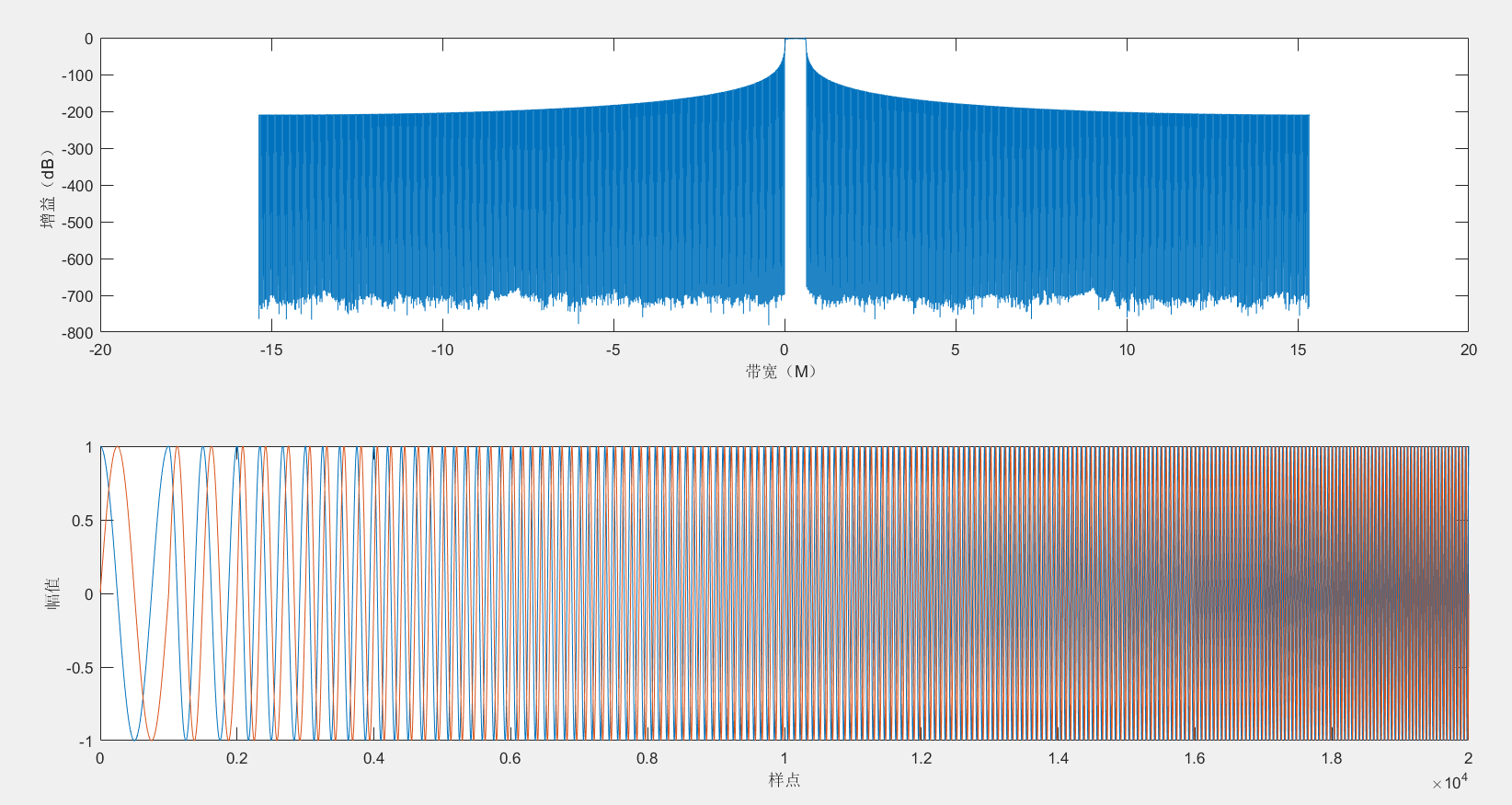% ==================================================
% 作者： jialf
% 时间： 2020/3/22
% 版本： V1
% =================FM线性调频仿真================
Fs = 30.72*10^6; %采样频率
period = 1000;   %每个频点的采样点数
t=1:period;
N=20;            %频点个数
fm_i = zeros(1,N*length(t));
fm_q = zeros(1,N*length(t));
for n=1:N
fm_i((n-1)*length(t)+1:(n-1)*length(t)+period) = cos(2*pi*t*n/period);
fm_q((n-1)*length(t)+1:(n-1)*length(t)+period) = sin(2*pi*t*n/period);
end
plot_xy(fm_i,fm_q,0,Fs); 

方式二：每个频率采样一个点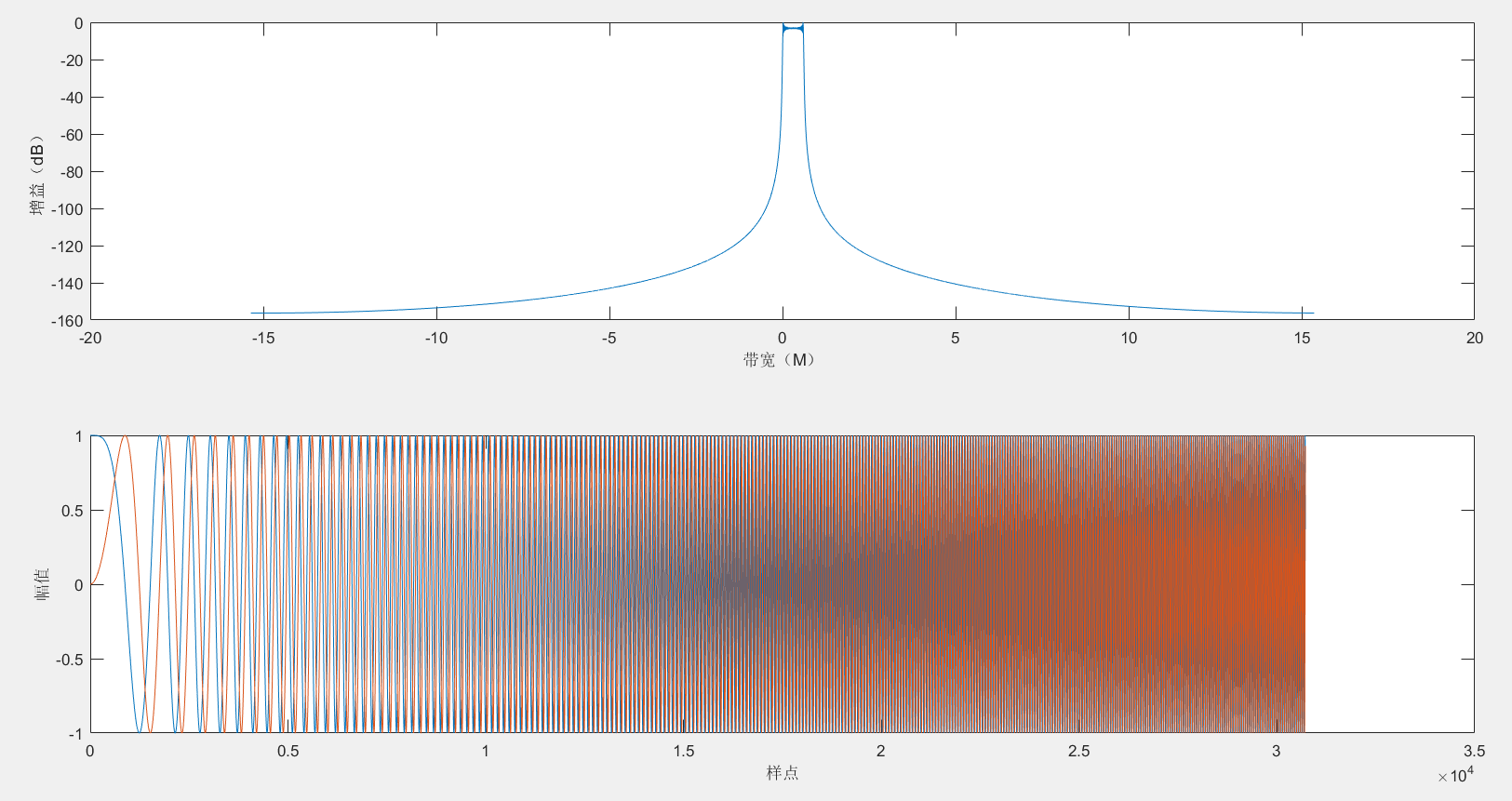% ==================================================
% 作者： jialf
% 时间： 2020/3/22
% 版本： V1
% =================FM线性调频仿真================
Fs = 30.72*10^6;  %采样频率
len = 30720;      %数据长度
f=0;              %频率变化间隔
n=0;
fm_i = zeros(1,len);
fm_q = zeros(1,len);
for t=0:1/Fs:(len-1)/Fs
n=n+1;
f=f+10;
fm_i(n) = cos(2*pi*f*t);
fm_q(n) = sin(2*pi*f*t);
end
plot_xy(fm_i,fm_q,0,Fs); 


展开全文• 根据不同的窗函数编制matlab程序产生不同的非线性调频信号 根据不同的窗函数编制matlab程序产生不同的非线性调频信号
• 线性调频信号可以获得较大的压缩比，有着良好的距离分辨率和径向速度分辨率，作为一种常用的脉冲压缩信号，已经广泛应用于高分辨率雷达领域。 　Matlab是美国MathWorks公司自20世纪80年代中期推出的数学软件，的...
• 线性调频信号可以获得较大的压缩比，有着良好的距离分辨率和径向速度分辨率，作为一种常用的脉冲压缩信号，已经广泛应用于高分辨率雷达领域。 　Matlab是美国MathWorks公司自20世纪80年代中期推出的数学软件，优秀...
• matlab线性调频信号产生算法，用于纤纤调频信号参数估计
背景：

1、高斯白噪声

在分析通信系统的抗噪声性能时，因为通信系统中常见的热噪声近似为白噪声，且热噪声的取值恰好服从高斯分布，
所以常用高斯白噪声作为通信信道中的噪声模型。其中噪声的统计均值为 0，统计方差为1，并且高斯白噪声的幅值-频次统计直方图服从正态分布。仿真结果如图所示，图1是高斯白噪声的时域波形，图2是高斯白噪声的归一化功率，图3是高斯白噪声的统计信息和幅值用来验证高斯白噪声分布的特点，其中程序的最后两行分别计算了噪声的均值和方差验证是否均值=0，方差=1，本次仿真的均值=5.3547e-4，接近于0，方差=0.9987，接近于1。

length = 1000000;
ff = 0:length-1;
noise = wgn(length,1,0);%生成100000*1个高斯白噪声，功率为0dBW（分贝瓦）
y1 = fft(noise,length);%采样点个数100000个
p1 = y1.*conj(y1);%conj()得到相应的复共轭数,y1.*conj(y1)就是模的平方
max_P=max(p1);%求功率的最大值
p1 =p1/(max_P);%除以最大值把功率谱归一化

subplot(2,2,1),plot(ff,noise(1:length)),axis([0 (length) -5 5]),xlabel('时间(s)'),ylabel('幅值(V)'),title('高斯白噪声波形');
subplot(2,2,2),plot(ff,p1(1:length)),axis([0 length 0 1]);xlabel('频率(Hz)');ylabel('功率');title('高斯白噪声归一化功率谱');
set(gca,'YTick',0:1:1);%设置逻辑值坐标轴为0和1,这样子就不会出现0-0.1-0.2^0.8-0.9-1的坐标，影响美观
subplot(2,2,3),hist(noise,40);axis([ -5 5 0 110000]);xlabel('幅值(V)');ylabel('频次');title('幅值-频次直方图');
mean_value = mean(noise)%计算噪声的均值，理论上应该是0
variance = var(noise)%计算噪声的方差，理论上应该为1，功率为0dBW（10*log1=0）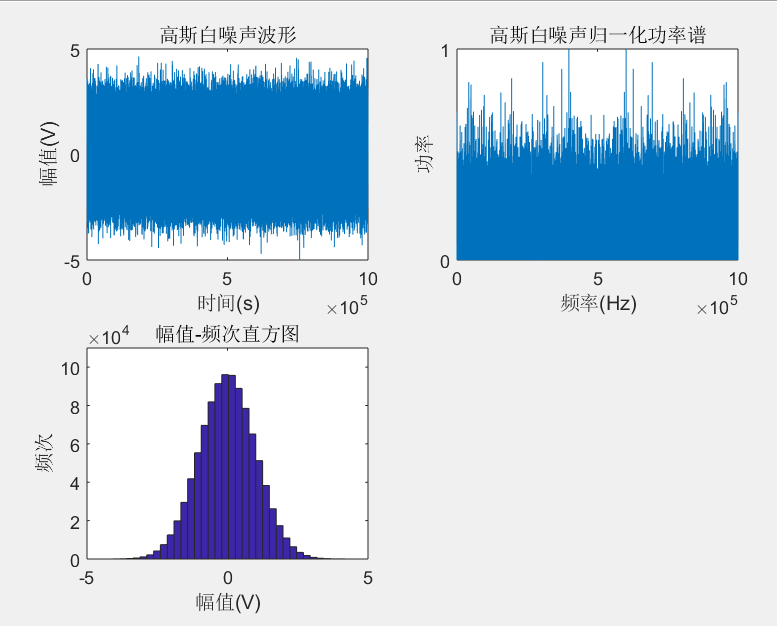2、噪声调幅信号

噪声调幅信号是是利用噪声作为调制信号，对载波信号进行AM调制，使得载波信号的振幅随基带噪声做随机变化，这里的噪声使用了上面的高斯白噪声进行调制。其中这里的上包络和下包络只是噪声信号的变形，不是噪声调幅信号真正的包络，只是为了便于理解调幅信号的本质所构造的辅助曲线。
噪声调幅信号的公式为：J ( t ) = ( U 0 + U n ( t ) ) cos ⁡ ( w j t + φ ) ， J(t)=\left(U_{0}+U_{n}(t)\right) \cos \left(w_{j} t+\varphi\right)，J(t)=(U0​+Un​(t))cos(wj​t+φ)，其中，U 0 U_{0}U0​是载波振幅，w j w_{j}wj​是载波角频率，U n ( t ) U_{n}(t)Un​(t)是基带噪声，φ φφ在[0,2π]内均匀分布。
通过分析图中噪声信号和噪声调幅干扰信号的波形与功率谱，可以发现噪声调幅干扰信号具有以下特点：

1) 噪声调幅干扰信号的功率谱由载波谱和对称旁瓣谱构成，旁瓣谱的形状与基带噪声功率谱的形状相似。

2) 噪声调幅干扰信号的带宽为基带噪声带宽的两倍。

fj=20e6;fs=4*fj; Tr=520e-6;
t1=0:1/fs:3*Tr-1/fs; N=length(t1);
u=wgn(1,N,0);%生成N*1个高斯白噪声，功率为0dBW（分贝瓦）
df1=fs/N;n=0:N/2;f=n*df1;
wp=10e6;ws=14e6;rp=1; rs=60;
[n1,wn1]=buttord(wp/(fs/2),ws/(fs/2),rp,rs);
[b,a]=butter(n1,wn1);
u1=filter(b,a,u);
p=0.1503*mean((u1.^2));
figure;subplot(2,2,1),plot(t1,u1),title('噪声信号波形'); axis([0,0.02e-4,-2,2]);xlabel('时间(s)');ylabel('幅度(V)');
subplot(2,2,2), j2=fft(u1);plot(f,10*log10(abs(j2(n+1)*2/N)));xlabel('频率(Hz)');ylabel('功率(dBW)');axis([0,4e7,-70,0]);title( '噪声信号功率谱');
u0=1;y=(u1+u0).*cos(2*pi*fj*t1+2);%噪声调幅信号的波形
u2=u1+u0;%上包络的波形
u3=-u0-u1;%下包络的波形
subplot(2,2,3), plot(t1,y,t1,u2,t1,u3),title( '噪声调幅信号时域波形'); axis([0,0.02e-4,-2,2]);xlabel('时间(s)');ylabel('幅度(V)');
subplot(2,2,4), J=fft(y);plot(f,10*log10(abs(J(n+1))));xlabel('频率(Hz)');ylabel('功率(dBW)');axis([0,4e7,-20,50]);title( '噪声调幅信号功率谱');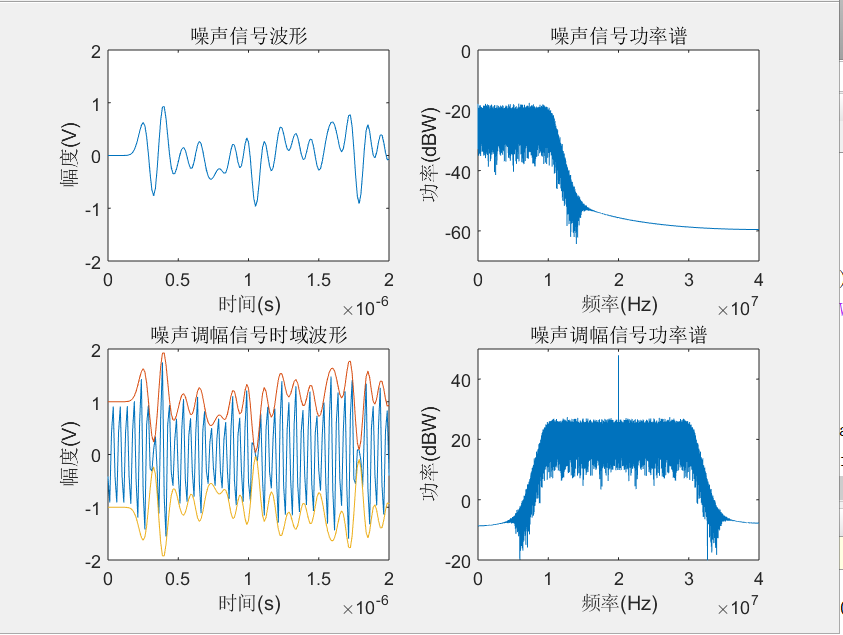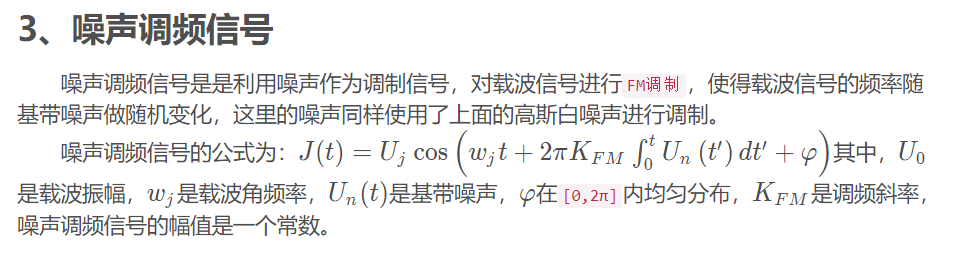uj=1;mf=2;wpp=10;
fj=20e6;fs=8*fj;Tr=520e-6;
t1=0:1/fs:3*Tr-1/fs;N=length(t1);
u=wgn(1,N,0);
wp=10e6;ws=16e6;rp=1;rs=60;
[n1,wn1]=buttord(wp/(fs/2),ws/(fs/2),rp,rs);
[b,a]=butter(n1,wn1);
u1=filter(b,a,u);
p=0.8503*mean((u1.^2)) ;
fj=20e6;fs=8*fj;Tr=520e-6;bj=5e6;
t1=0:1/fs:3*Tr-1/fs;N=length(t1);
u=wgn(1,N,wpp);
df1=fs/N;n=0:N/2;f=n*df1;
wp=10e6;ws=14e6;rp=1;rs=60;
[Nn,wn]=buttord(wp/(30e6/2),ws/(30e6/2),rp,rs);
[b,a]=butter(Nn,wn);
figure;subplot(2,2,1),plot(t1,u1),title('噪声信号波形');axis([0,2e-6,-2,2]);xlabel('时间(s)');ylabel('幅度(V)');
subplot(2,2,2),j2=fft(u1); plot(f,10*log10(abs(j2(n+1)*2/N)));xlabel('频率(Hz)');ylabel('功率（dBW）');axis([0,4e7,-20,50]);title( '噪声信号功率谱');axis([0,4e7,-80,0]);
i=1:N-1;ss=cumsum([0 u1(i)])
ss=ss*Tr/N;
y=uj*cos(2*pi*fj*t1+2*pi*mf*bj*ss*10);%uj=1 是输出的噪声调频信号的幅度  fj是调制信号中心频率是20M    增加调制指数*10 让波形明显
subplot(2,2,3), plot(t1,y),title( '噪声调频信号波形'),axis([0,2e-6,-1.5,1.5]);xlabel('时间(s)');ylabel('幅度(V)');
y=uj*cos(2*pi*fj*t1+2*pi*mf*bj*ss);%uj=1 是输出的噪声调频信号的幅度  fj是调制信号中心频率是20M
subplot(2,2,4),J=fft(y);plot(f,10*log10(abs(J(n+1))));axis([0,4e7,-20,60]);xlabel('频率(Hz)');ylabel('功率（dBW）');axis([0,4e7,-20,50]);title( '噪声调频信号功率谱')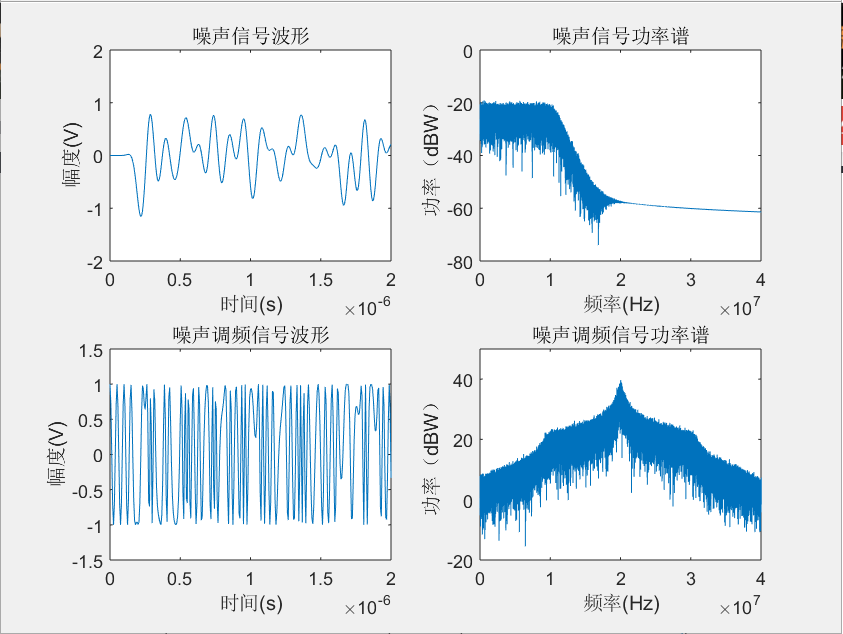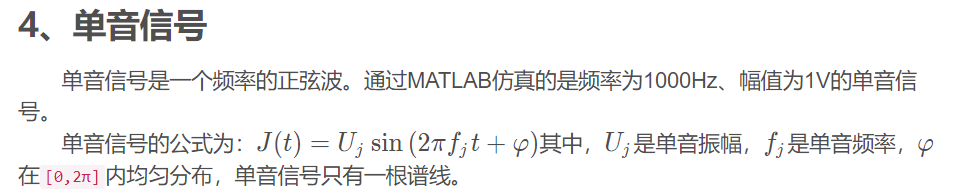Fs=20000;  %采样频率
N=20000;    %采样点
n=0:N-1;t=n/Fs;  %时间序列
fc=1000;  %载波信号频率
f=n*Fs/N;  %频率
Uc=1*sin(2*fc*pi*t);     %载波信号
C1=fft(Uc);             %对载波信号进行傅里叶变换
cxf=abs(C1);           %进行傅里叶变换
cxf=cxf/max(cxf);%归一化
subplot(3,1,1);plot(t,Uc);title('载波信号波形');xlabel('时间(s)');ylabel('幅度(V)');title('单音干扰信号波形');axis([0 0.009 -1 1]);
subplot(3,1,2); plot(f(1:N/2),cxf(1:N/2));title('载波信号频谱'); axis([0 2000 0 1]);xlabel('频率(Hz)');ylabel('功率');title('单音干扰信号归一化功率谱');
set(gca,'YTick',0:1:1);%设置功率谱坐标轴只有0和1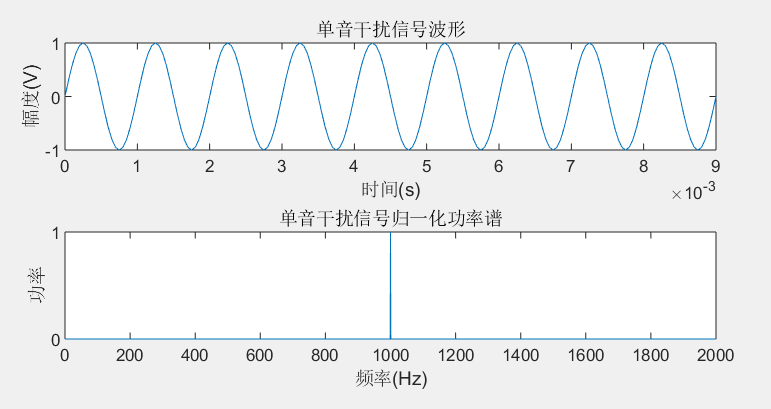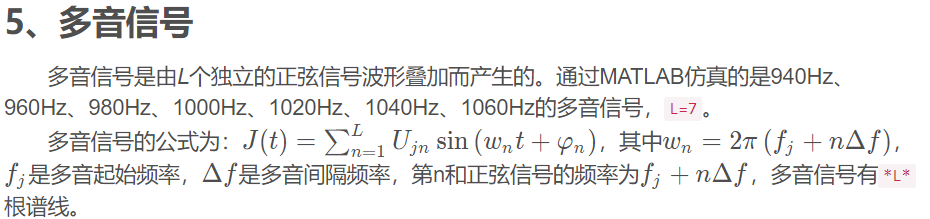Fs=200000;  %采样频率
N=200000;    %采样点
n=0:N-1;t=n/Fs;  %时间序列
A0=1;  %信号振幅
fc=1000;  %信号中间频率
f=n*Fs/N;  %信号步进频率
w0=2*fc*pi;
step=2*pi*50;
Uc=A0*cos(w0*t)+A0*cos((w0+step)*t)++A0*cos((w0+2*step)*t)++A0*cos((w0+3*step)*t)+A0*cos((w0-step)*t)++A0*cos((w0-2*step)*t)++A0*cos((w0-3*step)*t);%多音信号
C1=fft(Uc);      %对信号进行傅里叶变换
cxf=abs(C1);     %求绝对值
cxf=cxf/max(cxf);%归一化
subplot(2,1,1);plot(t,Uc);xlabel('时间(s)');ylabel('幅度(V)');title('多音信号波形');axis([0 0.1 -8 8]);
subplot(2,1,2);plot(f(1:N/2),cxf(1:N/2));title('载波信号频谱');axis([0 2000 0 1]);xlabel('频率(Hz)');ylabel('功率');title('多音信号归一化功率谱');
set(gca,'YTick',0:1:1);%设置功率谱坐标轴只有0和1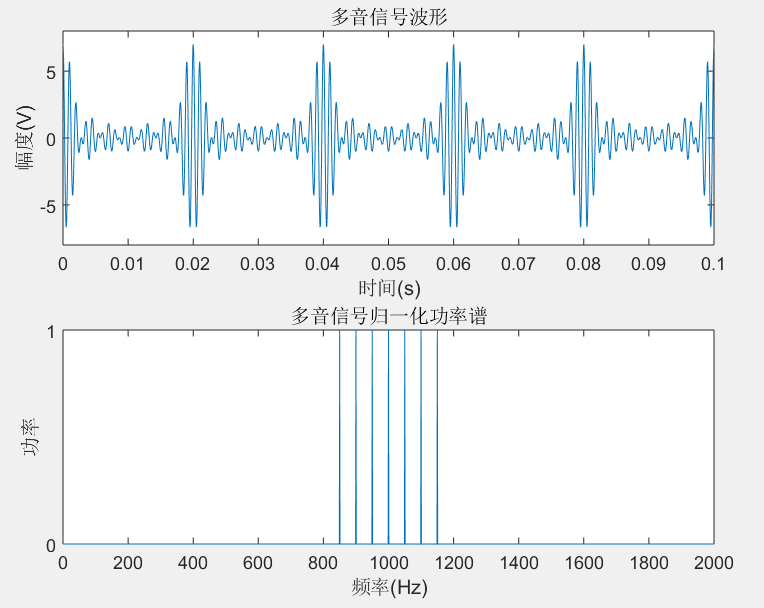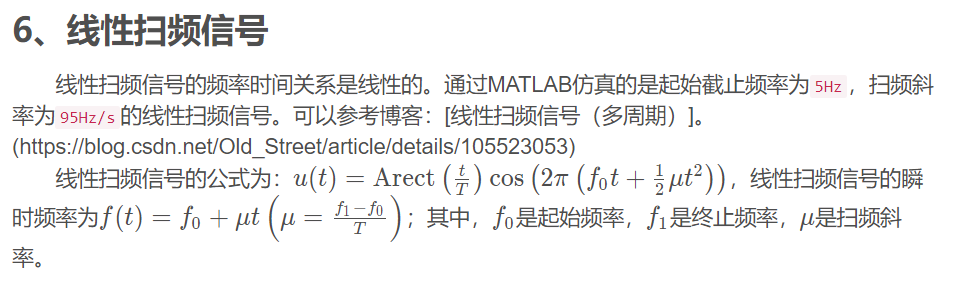t=0:0.00001:3-0.00001;%3对应3个周期，0.00001为精度
f0=5;%扫频起始频率
fe=100;%扫频截止频率
x=chirp(mod(t,1),f0,1,fe);%1代表的是单周期时间
subplot(3,1,1);plot(t,x);title('三个周期的线性扫频信号波形');xlabel('时间(s)');ylabel('幅度(V)');

ft=f0+(fe-f0)*mod(t,1);
subplot(3,1,2);plot(t,ft);title('线性扫频信号频率-时间图');xlabel('时间(s)');ylabel('频率(Hz)');

t=0:0.00001:1-0.00001;%求频谱时不能对多周期的求，对1个周期进行FFT
x=chirp(t,f0,1,fe);
C1=fft(x);     %对载波信号进行傅里叶变换
cxf=abs(C1);    %求绝对值
cxf=cxf/max(cxf);%归一化
subplot(3,1,3);plot(cxf); axis([0 150 0 1]);title('线性扫频信号归一化频谱');xlabel('频率(Hz)');ylabel('功率');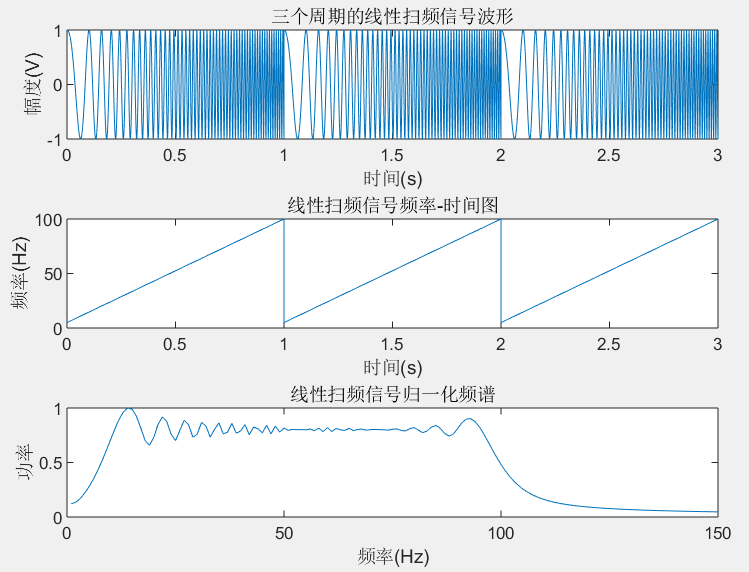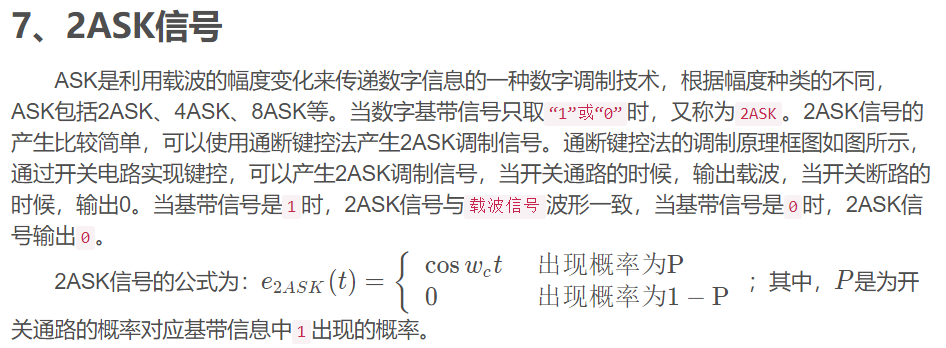N=10;%仿真10S的时间
xn=[];
x=[1 0 1 1 0 0 1 0 1 0];%每秒一个逻辑值，一共10个
t=0.001:0.001:N;%以1ms为步进
for i=1:N
if x(i)==1
xn(i*1000-999:i*1000)=ones(1,1000);
else
xn(i*1000-999:i*1000)=zeros(1,1000);
end
end
y=cos(2*pi*3*t);%载波波形 频率为3Hz
z=xn.*y;%载波调制
subplot(3,1,1);plot(xn);title(' 基带信号');xlabel('时间(ms)');ylabel('逻辑值');axis([0 10000 -0.2 1.2]);
set(gca,'YTick',-1:1:1);%设置逻辑值坐标轴只有0和1
subplot(3,1,2);plot(y);title(' 载波波形');xlabel('时间(ms)');ylabel('幅度(V)');axis([0 10000 -1 1]);
subplot(3,1,3);plot(z);title(' 2ASK信号');xlabel('时间(ms)');ylabel('幅度(V)');axis([0 10000 -1 1]);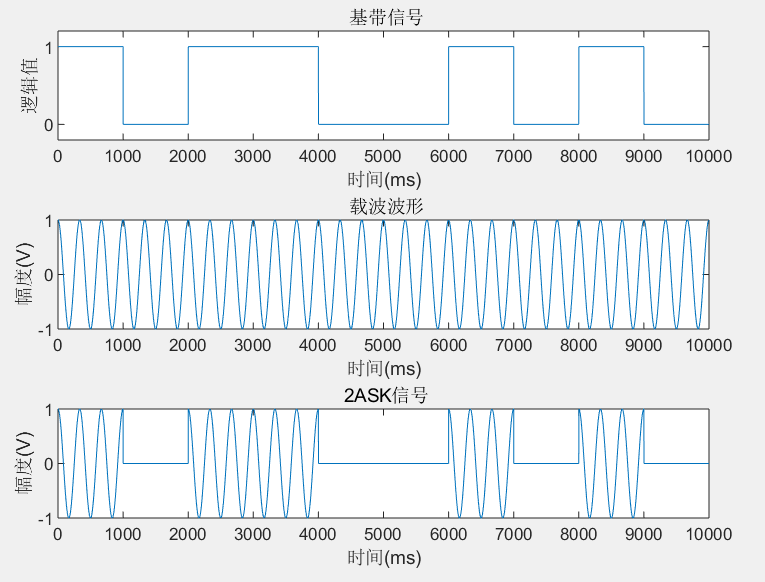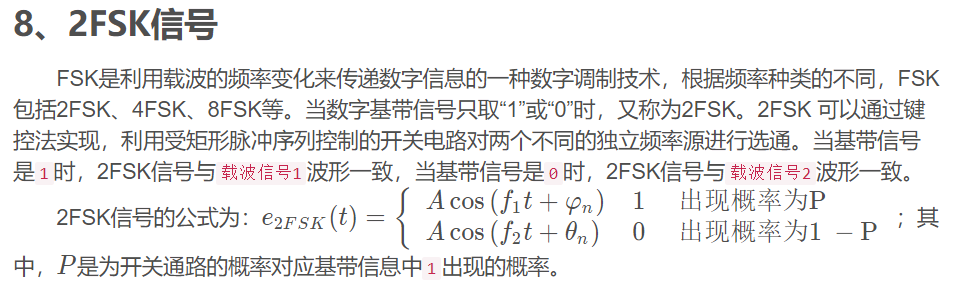N=10;%仿真10S的时间
xn=[];xn1=[];
x=[1 0 1 1 0 0 1 0 1 0];%%每秒一个逻辑值，一共10个
t=0.001:0.001:N;%以1ms为步进
for i=1:N
if x(i)==1
xn(i*1000-999:i*1000)=ones(1,1000);%xn都置为0
xn1(i*1000-999:i*1000)=zeros(1,1000);%xn1都置为1
else
xn(i*1000-999:i*1000)=zeros(1,1000);%xn都置为1
xn1(i*1000-999:i*1000)=ones(1,1000);%xn1都置为0
end
end
y=cos(2*pi*2*t);%载波波形1 频率为2Hz
y2=cos(2*pi*6*t);%载波波形2 频率为6Hz
F1=xn.*y; %加入载波1
F2=xn1.*y2; %加入载波2
e_fsk=F1+F2;%叠加
figure(1);heigth=160;width=160;set(gcf,'Position',[0 0 width/0.277 heigth/0.277]);%前面是图片在屏幕的位置，后面是图片大小为20*20
subplot(4,1,1);plot(xn);title(' 基带信号');xlabel('时间(ms)');ylabel('逻辑值');axis([0 10000 -0.2 1.2]);
set(gca,'YTick',-1:1:1);%设置逻辑值坐标轴只有0和1
subplot(4,1,2);plot(y);title(' 载波波形');xlabel('时间(ms)');ylabel('幅度(V)');axis([0 10000 -1 1]);
subplot(414);plot(e_fsk);title('2FSK信号');axis([0 10000 -1 1]);xlabel('时间(ms)');ylabel('幅度(V)');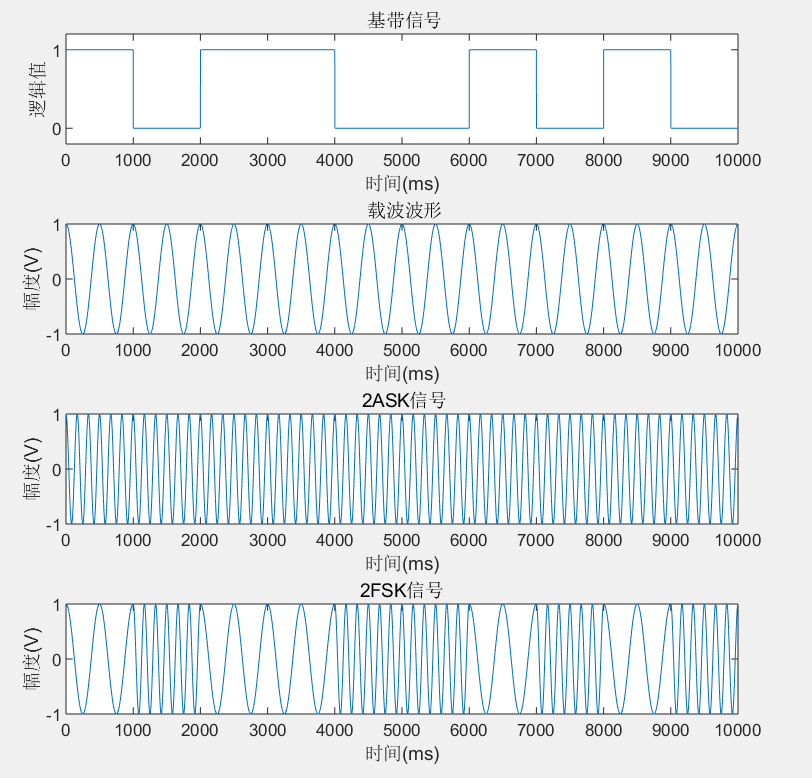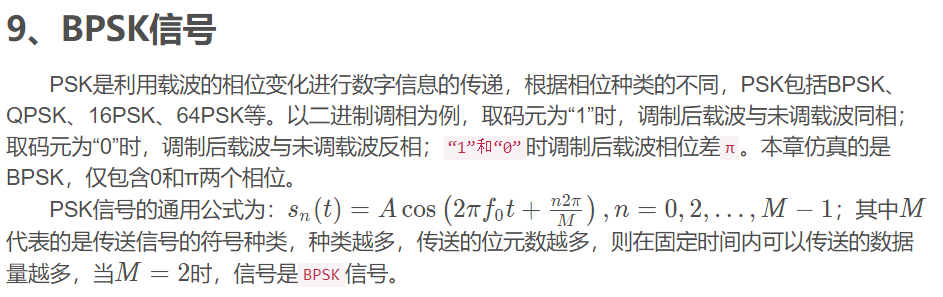N=10;%仿真10S的时间
xn=[];xn1=[];
x=[1 0 1 1 0 0 1 0 1 0];%每秒一个逻辑值，一共10个
t=0.001:0.001:N;%以1ms为步进
for i=1:N
if x(i)==1
xn(i*1000-999:i*1000)=ones(1,1000);
xn1(i*1000-999:i*1000)=ones(1,1000);%码元值都为1
else
xn(i*1000-999:i*1000)=-ones(1,1000);
xn1(i*1000-999:i*1000)=zeros(1,1000);%码元值都为0
end
end
y=sin(2*pi*1*t);%载波波形 频率为3Hz   与前面的不一样，为正弦波
z=xn.*y;%载波调制
subplot(3,1,1);plot(xn1);title(' 基带信号');xlabel('时间(ms)');ylabel('逻辑值');axis([0 10000 -0.2 1.2]);
set(gca,'YTick',-1:1:1);%设置逻辑值坐标轴只有0和1
subplot(3,1,2);plot(y);title(' 载波波形');xlabel('时间(ms)');ylabel('幅度(V)');axis([0 10000 -1 1]);
subplot(3,1,3);plot(z);title(' 2ASK信号');xlabel('时间(ms)');ylabel('幅度(V)');axis([0 10000 -1 1]);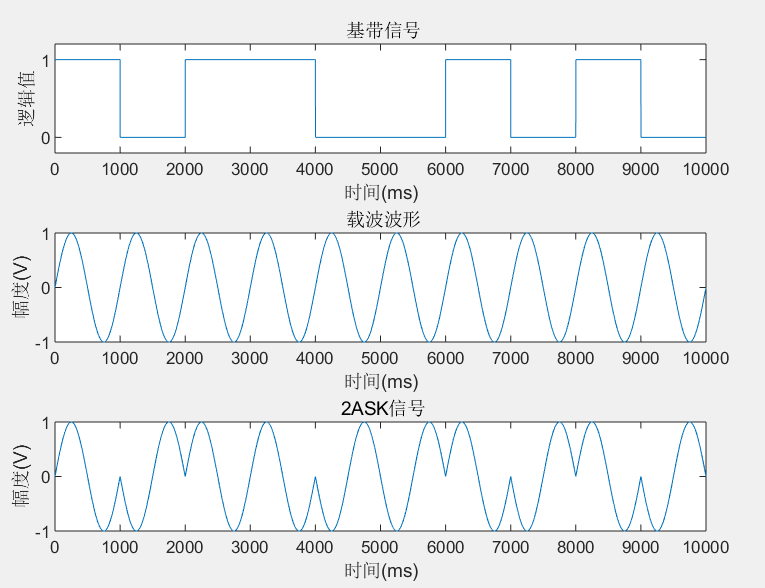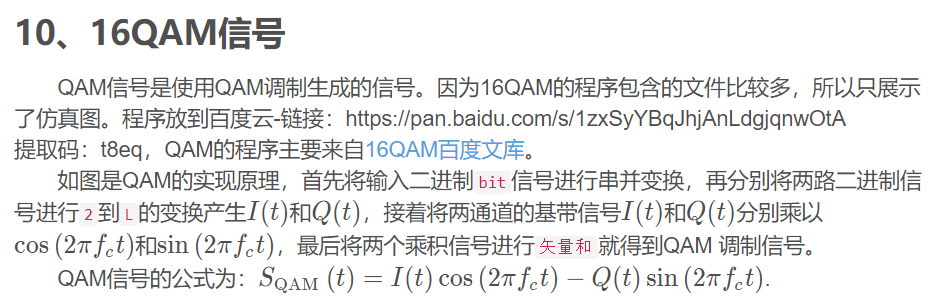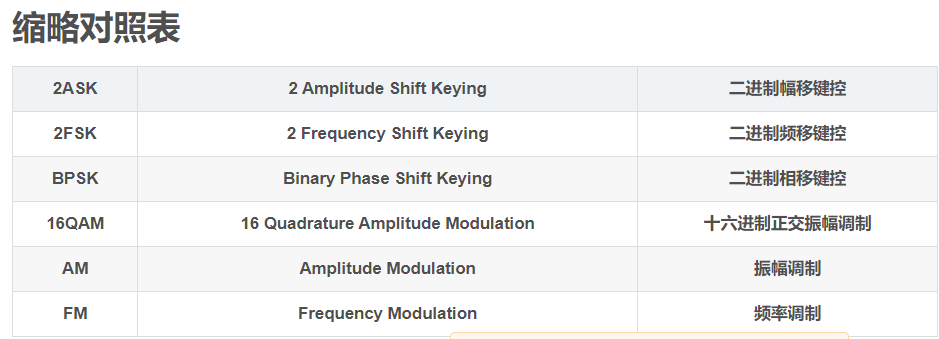展开全文机器学习 人工智能
• 线性调频信号，最大的优点就是波形的产生比较容易，此外该信号对多普勒频移不敏感，也就是说当存在多普勒频率偏移的时候，线性调频信号仍然能够应用。但LFM信号主要缺点是信号在匹配滤波后输出信号的旁瓣较高，第一...
线性调频信号，最大的优点就是波形的产生比较容易，此外该信号对多普勒频移不敏感，也就是说当存在多普勒频率偏移的时候，线性调频信号仍然能够应用。但LFM信号主要缺点是信号在匹配滤波后输出信号的旁瓣较高，第一旁瓣相对于主瓣为-13.2dB，无法满足实际的需要。从而发展出了加权网络技术，即在匹配滤波之后将信号通过一个加权网络来抑制旁瓣的影响。本章将重点介绍线性调频信号的脉冲压缩，并对其多普勒频率偏移的敏感性与旁瓣加权抑制技术进行研究，并通过MATLAB进行仿真分析。

在研究线性调频信号的脉冲压缩理论之前，我们首先通过原理图来说明线性调频脉冲压缩的基本原理。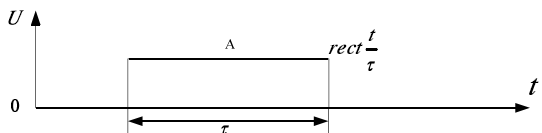图3.1a 输入信号的高频脉冲包络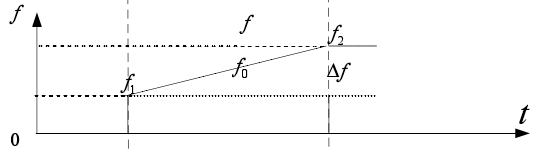图3.1b 线性调频过程中载频的调频特性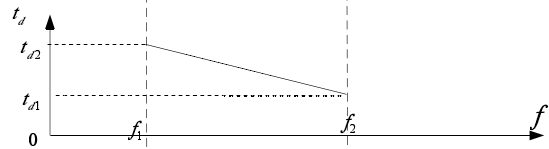图3.1c 压缩网络的频率延迟特性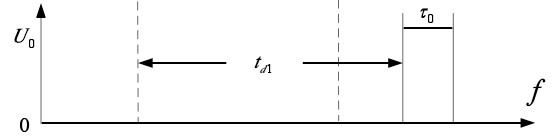图3.1d 压缩网络的输出脉冲包络

由上面的分析可知，线性调频信号通过匹配滤波器后，输出压缩脉冲的包络近似与sinc函数形状。最大的旁瓣电平为主瓣电平的-13.46dB，其他旁瓣按其离主瓣的间隔x按1/x规律衰减。图3.2给出了LFM信号的实部与虚部的基带波形。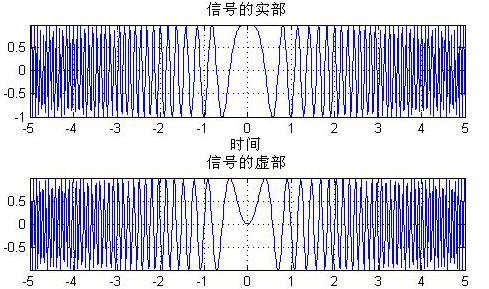图3.2 LFM信号仿真图（TB=300）

在实际系统中，接收到的信号往往是中频信号，首先需要对中频信号解调，还原出图3.2所示的I，Q两路信号。本文暂不考虑这个因素，本文主要在已知两路信号的前提下对系统进行仿真分析。

由前面的分析可知，线性调频信号通过匹配滤波器后，输出压缩脉冲的包络近似为sinc函数形状。其中最大的一对旁瓣为主瓣电平的-13.49dB，。在多目标环境中，这些旁瓣会埋没附近较小目标的主信号，引起目标丢失。图3.4的效果表面通过匹配滤波后的波形其旁瓣电平是比较大的。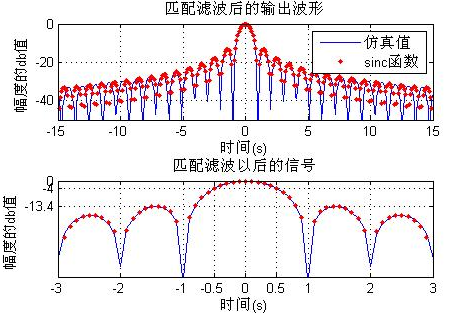图3.4 未加权前的信号仿真图

从仿真结果可以看到，当没有加权的时候，信号的旁瓣非常大，由图3.4第二个图可以看到，旁瓣幅度达到了主瓣的-13.4db。这会小回波的主峰可能造成严重干扰，降低雷达的性能。为了提高多目标的分辨能力，必须采用旁瓣抑制技术，即加权技术。加权技术就是对匹配滤波以后的信号进行窗操作。

下面通过一组雷达参数，来对本章的介绍进行系统的仿真与分析，设雷达的参数指标为：

表3.1 雷达参数指标

参数

指标

1

雷达发射信号参数

2

幅度

1.0

3

信号波形

线性调频信号

4

频带宽度

30MHz

5

脉冲宽度

10us

6

中心频率

109Hz

7

雷达接收方式

正交解调接收

8

距离门

10Km~15Km

根据前面的理论可知，线性调频后，其分辨率为：

四个目标，其相互之间间隔为1Km，所以是能够分别的，其仿真结果如下所示：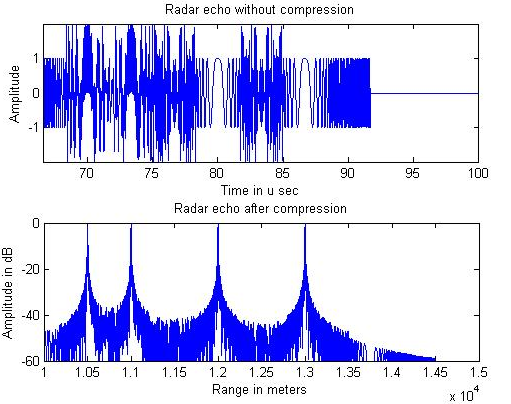图3.11 四目标线性调频后的仿真图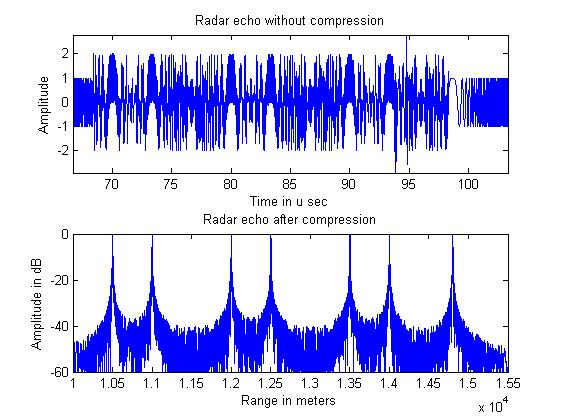图3.12 七目标线性调频后的仿真图

由此可见，线性调频脉冲压缩，其能够在一定范围之内对雷达信号进行分辨。当然，以上的仿真是在信号的功率相同的条件下进行的，如果接收到的信号功率不一致，如果两个目标相距比较远，系统仍然能够对系统进行分辨，但当弱信号在强信号的旁瓣范围之内，当信号的强度小于旁瓣电平的时候，强信号的旁瓣就会淹没掉弱信号的主瓣，从而无法识别信号。 图3.13的仿真将说明这个问题。

展开全文• 三、 基于DDS的线性调频信号产生由DDS原理可知通过控制输入的频率控制字即可控制输出的信号频率，我们可以通过控制频率控制字使DDS输出信号的频率线性变化，由此产生线性调频信号。由于在MATLAB中仿真能更清楚的...大侠好，欢迎来到FPGA技术江湖，江湖偌大，相见即是缘分。大侠可以关注FPGA技术江湖，在“闯荡江湖”、"行侠仗义"栏里获取其他感兴趣的资源，或者一起煮酒言欢。国际妇女节全称“联合国妇女权益和国际和平日”，在中国又称“国际劳动妇女节”、“三八节”和“三八妇女节”。现流行称为“女神节”、“女王节”，祝广大女神、女王，节日快乐。大侠好，今天由“82年的程序媛”本媛给大侠带来FPGA设计之基于DDS的线性调频信号的产生，后续本媛还会继续更新产品项目开发心得，学习心得等，欢迎大家持续关注，话不多说，上货。一、 DDS工作原理DDS(Direct Digital Synthesizer)技术是一种频率合成方法，其输出频率具有分辨率高、功耗低、频率切换速度快且频率切换时输出信号的相位连续等特点。为此在数字信号处理及硬件实现中有着很重要的作用。DDS的结构主要由相位累加器、波形存储器、数模转换器和低通滤波器等四个大的结构组成，其结构框图如下：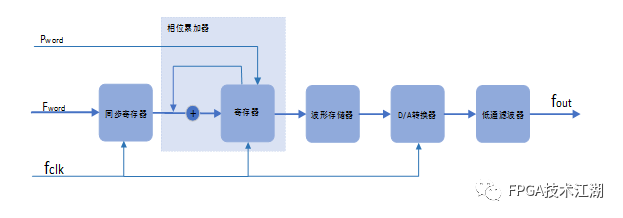其中，fclk为参考时钟，Pword为相位控制字，给定输出信号的初始相位值；Fword为频率控制字，用于控制输出信号的频率。DDS模块工作时，每来一个参考时钟，相位累加器就将频率控制字与寄存器输出的值累加，将相加后的值继续输入到寄存器中，这样构成一个循环，可以不停的对频率控制字进行累加。累加的值作为地址在波形存储器中通过查找地址所对应的幅值表，就可以完成其从相位到幅值之间的转化。在DDS模块中，输出频率的公式为：Fout = fclk/2*Fword从公式可以看出，DDS输出的频率由频率控制字Fword决定。当频率控制字变化时，输出频率也跟着变化，从而可以实现调频信号的产生。二、 线性调频信号线性调频信号是一种频率连续线性变化的信号，是一种常用的雷达信号。在雷达系统中常用做发射信号，由于其带宽较高且包络为矩形，故在接收机端可以实现脉冲压缩得到较高的距离分辨力。线性调频信号表达式为：S(t)=Acos(2πf0 + πut^2) (0≤t≤τ)；相位对 t 求导得到频率： f=f0+ut；其中：f0为起始频率，u为调频斜率，τ为脉冲宽度，B=uτ为信号的带宽。由公式可以看出，S(t)的频率随时间变化线性变化，初始值为f0，斜率为u。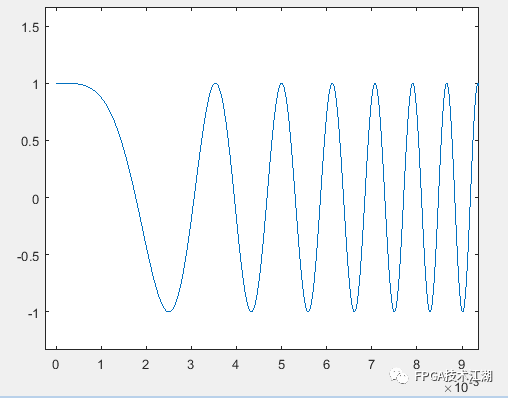三、 基于DDS的线性调频信号产生由DDS原理可知通过控制输入的频率控制字即可控制输出的信号频率，我们可以通过控制频率控制字使DDS输出信号的频率线性变化，由此产生线性调频信号。由于在MATLAB中仿真能更清楚的了解信号的频谱，故本实验采用在system generator中搭建模型的方式，仿真正确后生成IP核的加入到工程中。首先，我们需要确定线性调频信号的相关参数，即模型的输入包括采样率fs、信号的带宽B、斜率选择ratio以及初始相位frq_first等。整体模型框图如下图所示：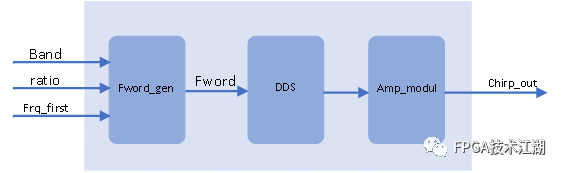首先根据带宽及采样率通过fword_gen模块产生线性的DDS的频率控制字，并且给出初始相位和斜率选择；将计算得到的频率控制字输出给DDS，产生频率在Band带宽内线性变化的余弦信号；最后通过幅度调制模块对输出信号的振幅进行调整，得到一个chirp信号。DDS模块调用xilinx的IP核DDS compiler6.0，可以双击IP核进行配置其系统时钟、相位位宽、输出信号位宽等。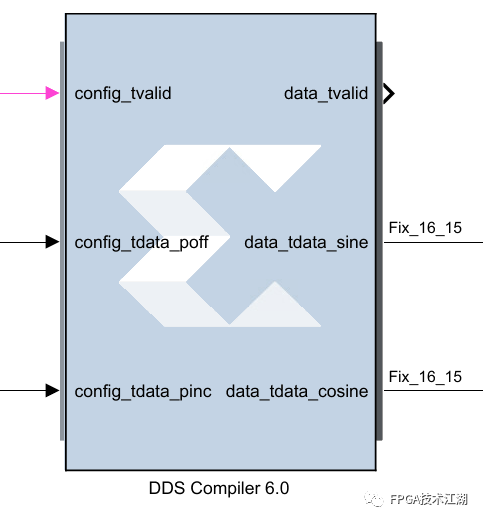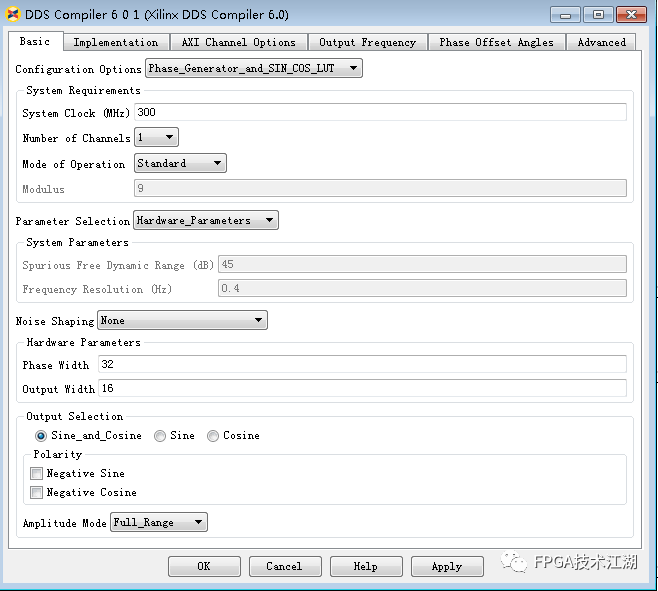其中poff为相位控制字，pinc为频率控制字。需要注意的是，在搭建模型调用IP核时，输入的相位控制字和频率控制字均需要为小数，可以通过调用reinterpret模块，将fword_gen模块生成的频率控制字转换成小数后输入给DDS IP核。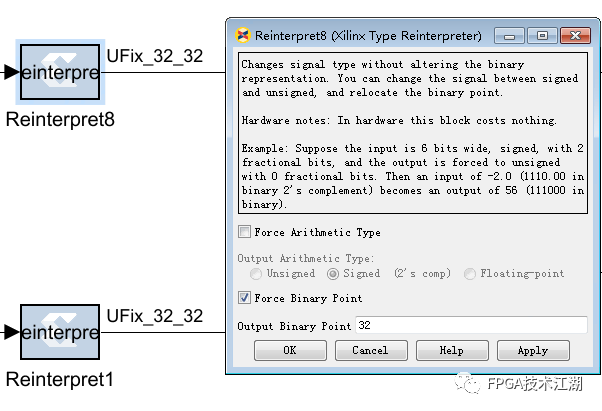最后通过在matlab环境下仿真得到的chirp信号如下图所示：分别为信号实部、虚部以及初始相位375M、带宽为500M的信号频谱图。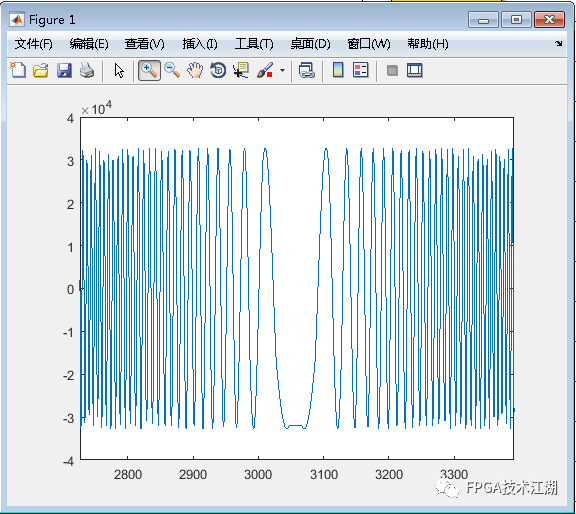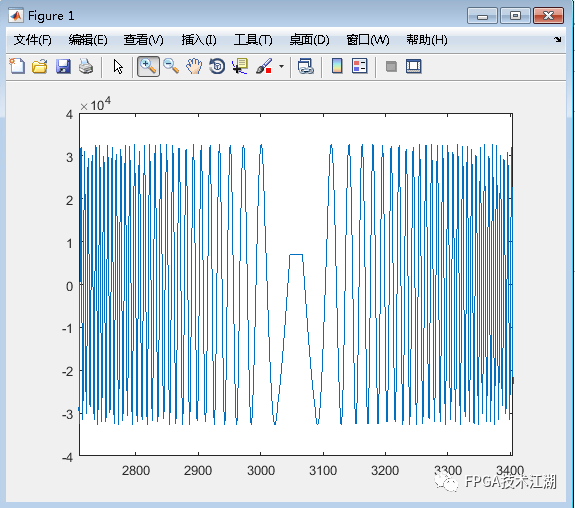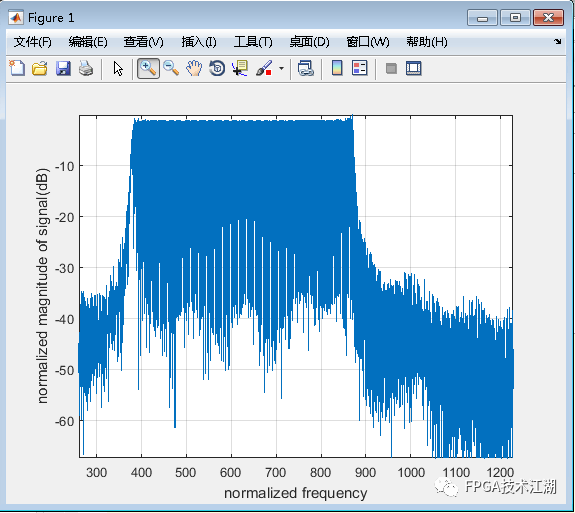今天本媛就说到这里，后续继续和大侠一起分享，欢迎关注貌美如花的“82年的程序媛”本媛，江湖偌大，继续闯荡，加油！END后续会持续更新，带来Vivado、 ISE、Quartus II 、candence等安装相关设计教程，学习资源、项目资源、好文推荐等，希望大侠持续关注。大侠们，江湖偌大，继续闯荡，愿一切安好，有缘再见！FPGA技术江湖广发江湖帖无广告纯净模式，给技术交流一片净土，从初学小白到行业精英业界大佬等，从军工领域到民用企业等，从通信、图像处理到人工智能等各个方向应有尽有，QQ微信双选，FPGA技术江湖打造最纯净最专业的技术交流学习平台。FPGA技术江湖微信交流群加群主微信，备注职业+方向+名字进群FPGA技术江湖QQ交流群备注地区+职业+方向+名字进群展开全文• 三、 基于DDS的线性调频信号产生由DDS原理可知通过控制输入的频率控制字即可控制输出的信号频率，我们可以通过控制频率控制字使DDS输出信号的频率线性变化，由此产生线性调频信号。由于在MATLAB中仿真能更清楚的...
• %线性调频信号产生matlab仿真代码 %...................................................................................% clear all; clc; %%信号的参数设置 T=10e-6; %pulse duration10us B=30e6; .....
• %线性调频信号产生matlab仿真代码 %...................................................................................% clear all; clc; %%信号的参数设置 T=10e-6; %pulse duration10us B=30e6; ...
• %线性调频信号分为IQ两路时域图和频谱的产生matlab仿真代码 %注意：复信号，要保证采样频率fs&gt;带宽B，频谱才不会混叠。实信号则需要fs&gt;2B。 %为了防止混叠：Fs&gt;=2f0 %...........................
• 近程模式下的单脉冲信号产生，频谱图,模糊函数图,模糊度等高线图，自相关函数图和多普勒敏感度Matlab代码
• ## MATLAB LFCM雷达调频法测距

千次阅读 多人点赞 2021-04-21 20:56:03
雷达原理老师留的作业，干了一整天，收获蛮大，拿word写的报告，夜已经深了（21点），懒得转成LaTeX了，就直接截图放上来了。 一、问题提出 LFCM（线性调频连续波）雷达。...2.线性调频信号（LFM）产fft
• 本文研究了线性调频信号脉冲压缩的副瓣抑制问题。由于在压缩过程中，会在窄脉冲两侧不可避免地产生以辛格函数为包络的副瓣，副瓣的存在会大大降低多目标分辨能力，故采用了副瓣抑制的加权处理方法即通过海明加权，使...
• ## MATLAB学习笔记之chirp信号的产生

千次阅读 多人点赞 2020-02-08 16:56:10
chirp信号即线性调频信号，是指瞬时频率随时间呈线性变化的信号。 chirp信号的matlab程序实现方法如下： 1.可利用matlab自带的chirp函数生成。 chirp（t，f0，T，f1）； T表示脉冲宽度，f0表示起始频率，f1表示...
• 单周期线性调频信号及频谱分析；多周期线性调频信号及频谱分析；STFT变换；噪声调频干扰信号的产生和功率谱；
• MATLAB中，产生线性调频扫频信号的函数chirp，其调用格式如下： y=chirp(t,f0,t1,f1)：产生一个线性(频率随时间线性变化)信号，其时间轴由数组t定义。时刻t0的瞬时频率为f0时刻，时刻t1的瞬时频率为f1。默认情况...
• ## 雷达信号matlab仿真

热门讨论 2010-10-15 20:52:24
该文献为雷达信号的MATLAB仿真，内容涉及线性调频信号产生，模糊函数，脉冲压缩，相参积累，信噪比
• MATLAB产生各种LPI雷达信号，包括线性调频信号、巴克码信号、弗兰克码信号、P1、2、3、4码等信号
• 该楼层疑似违规已被系统折叠隐藏此楼查看此楼小女子 路遇强悍的毕业设计 来到贴吧 求...在此给出一点程序 看可不可以帮助到大家线性调频信号产生程序T=10e-6; %pulse duration10usB=30e6; %chirp frequency modula...
• 完整的下变频matlab仿真程序，包括：1产生线性调频信号； 2对线性调频信号的时频谱图分析 ；3进行数字下变频 ； 4进行低通滤波，处理I路和Q路信号，滤波后输出，画图分析。
• ex9_2 载波10MHz，带宽2MHz的线性调频信号及其频谱图 ex9_3 产生7位巴克码编码的二相码 ex9_4 产生7位巴克码和线性调频的混合调制信号 ex9_5 瑞利分布实现程序 ex9_6 瑞利分布＋杂波 ex9_7 相关对数正态分布杂波 ex9...
• %线性调频信号(LFM)的产生 T = 2e-6; %脉宽 B = 40e6; %信号带宽 K = B/T; %调频斜率 Fs = 48e6; Ts = 1/Fs; %采样频率及采样间隔； N = round(T/Ts); %生成N个点 t = linspace(-T/2,T/2,N) fc = 20.0e9; %中心频率 ...图像处理
• ex9_2 载波10MHz，带宽2MHz的线性调频信号及其频谱图 ex9_3 产生7位巴克码编码的二相码 ex9_4 产生7位巴克码和线性调频的混合调制信号 ex9_5 瑞利分布实现程序 ex9_6 瑞利分布＋杂波 ex9_7 相关对数正态分布杂波 ex9...
• 1.波形产生函数 square函数：产生方波 sawtooth函数：三角波 ...chirp函数：产生线性调频余弦信号。。 2.傅里叶变换函数 fft函数： fftshift函数： ifft函数：傅里叶反变换 3.滤波器分析...# matlab产生线性调频信号matlab 订阅